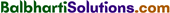By going through these Maharashtra State Board 12th Science Chemistry Notes Chapter 4 Chemical Thermodynamics students can recall all the concepts quickly.

## Maharashtra State Board 12th Chemistry Notes Chapter 4 Chemical Thermodynamics

→ W = -P (V2 – V1) = -PΔV (For expansion)

→ W = P (V2 – V1) = PΔV (For compression)

→ Wmax = -2.303 nRT log10 $$\frac{V_{2}}{V_{1}}$$

→ Wmax= -2.303 nRT log10 $$\frac{P_{1}}{P_{2}}$$→ ΔU = q + W

→ H = U + PV

→ ΔH = ΔU + PΔV

→ ΔH = ΔU + ΔnRT

→ Hess’s law : ΔH = ΔH1 + ΔH2 + ΔH3

→ ΔS = $$\frac{q_{\mathrm{rev}}}{T}=\frac{\Delta H}{T}$$

→ G = H – TS

→ ΔG = ΔH – TΔS

→ ΔG°= – 2.303 RTlog10K

→ (i) ΔG = 0, the system is at equilibrium
(ii) ΔG < 0, the process is spontaneous
(iii) ΔG > 0, the process is non-spontaneous.